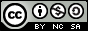- 目录 -
LeetCode 647 回文子串(Manacher)

输入："abc"



输入："aaa"



• 输入的字符串长度不会超过 1000 。

## 思路

• p[i] 代表以第 i 个位置为中心的最长回文半径（包括 i
• p[i]-1 代表，原串中以第 i 个位置为中心的最长回文串长度
• pos 代表当前最右边为 max_right 元素时的回文串中心
• max_right 代表当前访问到的所有回文子串，所能触及的最右一个字符的位置。

## 代码

  1 2 3 4 5 6 7 8 9 10 11 12 13 14 15 16 17 18 19 20 21 22 23 24 25 26 27 28 29 30 31 32 33 34 35 36  class Solution { public: vector p; int pos, max_right; string init(string s) { string ans = "#"; for (char ch : s) ans = ans + ch + '#'; return ans; } int countSubstrings(string s) { int ans = 0; s = init(s); p.resize(s.size()); pos = 0, max_right = 0; for (int i = 0; i < s.size(); i++) { if (i < max_right) p[i] = min(p[2 * pos - i], max_right - i); else p[i] = 1; while (i - p[i] >= 0 && i + p[i] < s.size() && s[i - p[i]] == s[i + p[i]]) p[i]++; if (p[i] + i - 1 > max_right) { max_right = p[i] + i - 1; pos = i; } ans += ((p[i] - 1) + 1) / 2; } return ans; } };